JEE  >  Subjective Type Questions: Solutions- 2 | JEE Advanced

# Subjective Type Questions: Solutions- 2 | JEE Advanced - Notes | Study Chemistry 35 Years JEE Main & Advanced Past year Papers - JEE

 1 Crore+ students have signed up on EduRev. Have you?

7. The vapour pressure of ethanol and methanol are 44.5 mm and 88.7 Hg respectively. An ideal solution is formed at the same temperature by mixing 60 g of ethanol with 40 g of methanol. Calculate the total vapour pressure of the solution and the mole fraction of methanol in the vapour. (1986 - 4 Marks)

Ans : 66.17 mm, 0.65

Solution :
TIPS/Formulae :
Ptotal = pA + pB
Molecular weight of CH3OH = 12 + 3 + 16 + 1 = 32
Molecular weight of C2H5OH= 24 + 5 + 16 + 1 = 46
According to Raoult’s law
Ptotal = p1 + p2
where Ptotal = Total vapour pressure of the solution
p1 = Partial vapour pressure of one component
p2 = Partial vapour pressure of other component
Again, p1 = Vapour pressure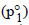× mole fraction
Similarly, p2 = Vapour pressure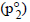x mole fraction

Mole fraction of CH3OH =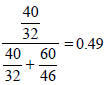Mole fraction of ethanol =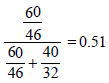NOTE THIS STEP : Thus now let us first calculate the partial vapour pressures, i.e., p1 and p2 of the two component.
Partial vapour pressure of CH3OH(p1)= 88.7 × 0.49 = 43.48 mm
Partial vapour pressure of C2H5OH(p2) = 44.5 × 0.51 = 22.69 mm
∴ Total vapour pressure of the solution = 43.48 + 22.69 mm = 66.17 mm
Mole fraction of CH3OH in vapour =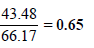8. The vapour pressure of a dilute aqueous solution of glucose (C6H12O6) is 750 mm of mercury at 373 K. Calculate (i) molality and (ii) mole fraction of the solution. (1989 - 3 Marks)

Ans : 0.7503 mol/kg, 0.9868

Solution :
TIPS/Formulae :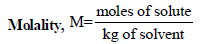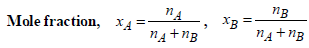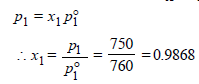x2 (solute) = 1 – 0.9868 = 0.0132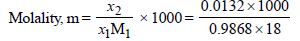= 0.7503 mol kg–1

9. The vapour pressure of pure benzene at a certain temperature is 640 mm Hg. A non-volatile non-electrolyte solid weighing 2.175 g is added to 39.0 g of benzene. The vapour pressure of the solution is 600 mm Hg. What is the molecular weight of the solid substance? (1990 - 3 Marks)

Ans : 65.25

Solution :
TIPS/Formulae :

According to Raoult’s law,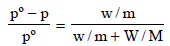Here, pº = 640 mm
p = 600 mm
w= 2.175 g
= 39.0
m = ?
M = 78
Substituting the various values in the above equation for Roult's law :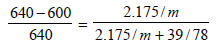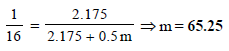10. The degree of dissociation of calcium nitrate in a dilute aqueous solution, containing 7.0 g. of the salt per 100 gm of water at 100ºC is 70%. If the vapour pressure of water at 100ºC is 760 mm, calculate the vapour pressure of the solution. (1991 - 4 Marks)

Ans : 746.3 mm Hg

Solution :
TIPS/Formulae : First find moles of Ca(NO3)2 and water.
Then use the expression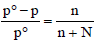to find vapour pressure of solution.
Let initially 1 mole of Ca(NO3)2 is taken
Degree of dissociation of Ca(NO3)2 = 70/100 = 0.7
Ionisation of Ca(NO3)2 can be represented as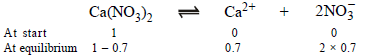∴ Total number of moles in the solution at equilibrium = (1 – 0.7) + 0.7 + 2 × 0.7 = 2.4

No. of moles when the solution contains 1 gm of calcium nitrate instead of 1 mole of the salt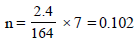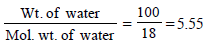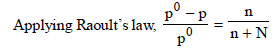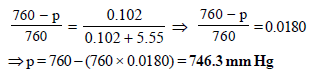11. Addition of 0.643 g of a compound to 50 ml. of benzene (density : 0.879 g/ml.) lowers the freezing point from 5.51ºC to 5.03ºC. If Kf for benzene is 5.12 K kg mol–1, calculate the molecular weight of the compound. (1992 - 2 Marks)

Ans : 156.056

Solution :

TIPS/Formulae :
Given Wt. of benzene (solvent),
W = Volume × density = 50 × 0.879 = 43.95 g
Wt. of compound (solute), w = 0.643 g
Mol. wt. of benzene, M = 78; Mol. wt. of solute, m = ?
Depression in freezing point, DTf = 5.51 – 5.03 = 0.48
Molal freezing constant, Kf = 5.12
Now we know that,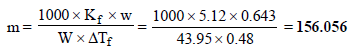12. What weight of the non-volatile solute, urea (NH2 – CO – NH2) needs to be dissolved in 100g of water, in order to decrease the vapour pressure of water by 25%? What will be the molality of the solution? (1993 - 3 Marks)

Ans : 18.52 m

Solution :

TIPS/Formulae :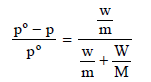Here, w and m are wt. and molecular wt. of solute, W and M are wt. and molecular weight of solvent
p = Pressure of solution; pº = Normal vapour pressure
Let the initial (normal) pressure (pº) = p

∴ Pressure of solution =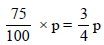m = 60, M = 18, W = 100 gm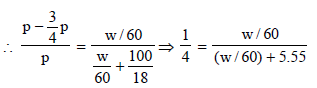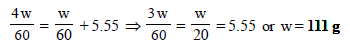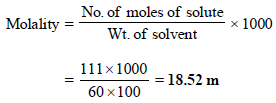13. The molar volume of liquid benzene (density=0.877 g mL–1) increases by a factor of 2750 as it vaporises at 20°C and that of liquid toluene   (density=0.867 g mL–1) increases by a factor of 7720 at 20°C. A solution of benzene and toluene at 20°C has a vapour pressure of 46.0 Torr. Find the mole fraction of benzene in the vapour above the solution. (1996 - 3 Marks)
Ans : 0.73

Solution :

TIPS/Formulae :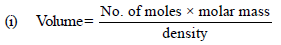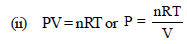Volume of 1 mole of liq. benzene =78/0.877
Volume of 1 mole of toluene = 92/0.867

In vapour phase,
At 20°C, for 1 mole of benzene,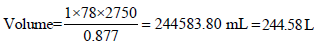Similarly for 1mole of toluene,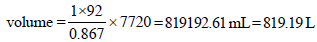As we know that, PV = nRT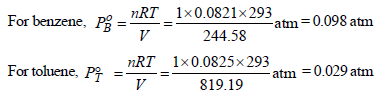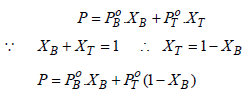Total vapour-pressure = 46 torr = 46/760 = 0.060  atm
Thus, 0.060 = 0.098 XB + 0.029 (1 – XB)
⇒ 0.060 = 0.098 XB + 0.029 – 0.029 XB
⇒ 0.031 = 0.069 XB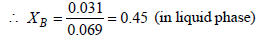XB + XT = 1
XT = 1– 0.45 = 0.55 (in liquid phase)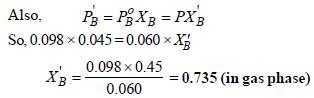The document Subjective Type Questions: Solutions- 2 | JEE Advanced - Notes | Study Chemistry 35 Years JEE Main & Advanced Past year Papers - JEE is a part of the JEE Course Chemistry 35 Years JEE Main & Advanced Past year Papers.
All you need of JEE at this link: JEE

## Chemistry 35 Years JEE Main & Advanced Past year Papers

142 docs|66 tests

## Chemistry 35 Years JEE Main & Advanced Past year Papers

142 docs|66 tests

Track your progress, build streaks, highlight & save important lessons and more!

,

,

,

,

,

,

,

,

,

,

,

,

,

,

,

,

,

,

,

,

,

;